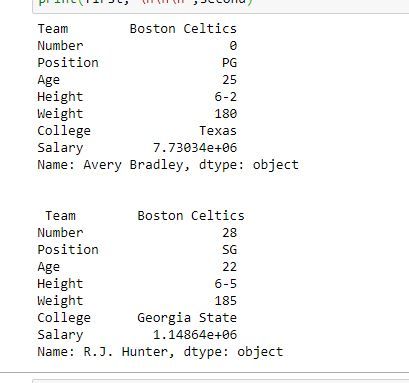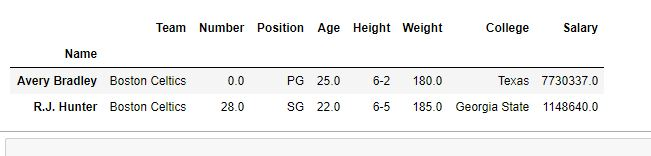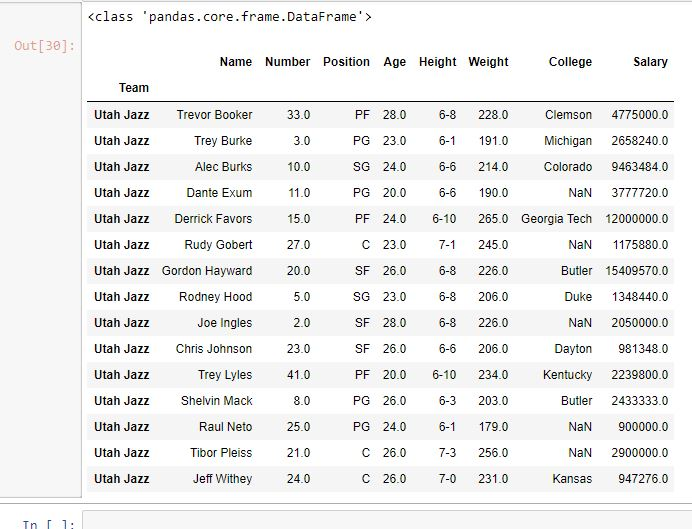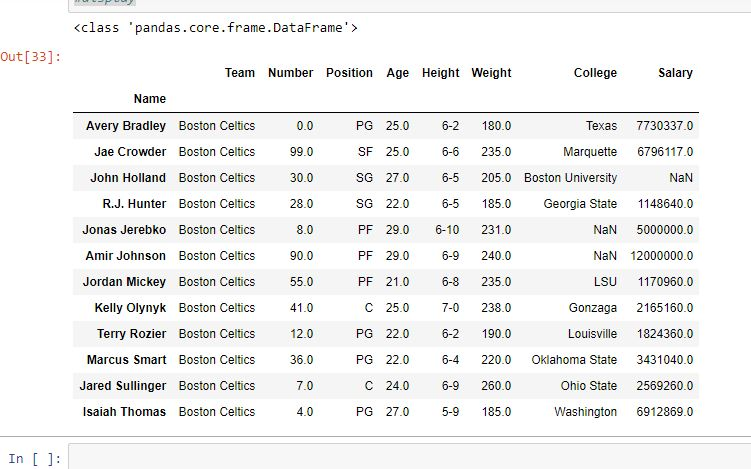# Python | Pandas Extracting rows using .loc[]

Python is a great language for doing data analysis, primarily because of the fantastic ecosystem of data-centric Python packages. Pandas is one of those packages and makes importing and analyzing data much easier.

Pandas provide a unique method to retrieve rows from a Data frame. `DataFrame.loc[]` method is a method that takes only index labels and returns row or dataframe if the index label exists in the caller data frame.

Syntax: pandas.DataFrame.loc[]

Parameters:
Index label: String or list of string of index label of rows

Return type: Data frame or Series depending on parameters

Example #1: Extracting single Row

In this example, Name column is made as the index column and then two single rows are extracted one by one in the form of series using index label of rows.

 `# importing pandas package ` `import` `pandas as pd ` ` `  `# making data frame from csv file ` `data ``=` `pd.read_csv(``"nba.csv"``, index_col ``=``"Name"``) ` ` `  `# retrieving row by loc method ` `first ``=` `data.loc[``"Avery Bradley"``] ` `second ``=` `data.loc[``"R.J. Hunter"``] ` ` `  ` `  `print``(first, ``"\n\n\n"``, second) `

Output:
As shown in the output image, two series were returned since there was only one parameter both of the times.Example #2: Multiple parameters

In this example, Name column is made as the index column and then two single rows are extracted at the same time by passing a list as parameter.

 `# importing pandas package ` `import` `pandas as pd ` ` `  `# making data frame from csv file ` `data ``=` `pd.read_csv(``"nba.csv"``, index_col ``=``"Name"``) ` ` `  `# retrieving rows by loc method ` `rows ``=` `data.loc[[``"Avery Bradley"``, ``"R.J. Hunter"``]] ` ` `  `# checking data type of rows ` `print``(``type``(rows)) ` ` `  `# display ` `rows `

Output:
As shown in the output image, this time the data type of returned value is a data frame. Both of the rows were extracted and displayed like a new data frame.Example #3: Extracting multiple rows with same index

In this example, Team name is made as the index column and one team name is passed to .loc method to check if all values with same team name have been returned or not.

 `# importing pandas package ` `import` `pandas as pd ` ` `  `# making data frame from csv file ` `data ``=` `pd.read_csv(``"nba.csv"``, index_col ``=``"Team"``) ` ` `  `# retrieving rows by loc method ` `rows ``=` `data.loc[``"Utah Jazz"``] ` ` `  `# checking data type of rows ` `print``(``type``(rows)) ` ` `  `# display ` `rows `

Output:
As shown in the output image, All rows with team name “Utah Jazz” were returned in the form of a data frame.Example #4: Extracting rows between two index labels

In this example, two index label of rows are passed and all the rows that fall between those two index label have been returned (Both index labels Inclusive).

 `# importing pandas package ` `import` `pandas as pd ` ` `  `# making data frame from csv file ` `data ``=` `pd.read_csv(``"nba.csv"``, index_col ``=``"Name"``) ` ` `  `# retrieving rows by loc method ` `rows ``=` `data.loc[``"Avery Bradley"``:``"Isaiah Thomas"``] ` ` `  `# checking data type of rows ` `print``(``type``(rows)) ` ` `  `# display ` `rows `

Output:
As shown in the output image, all the rows that fall between passed two index labels are returned in the form of a data frame.My Personal Notes arrow_drop_upCheck out this Author's contributed articles.

If you like GeeksforGeeks and would like to contribute, you can also write an article using contribute.geeksforgeeks.org or mail your article to contribute@geeksforgeeks.org. See your article appearing on the GeeksforGeeks main page and help other Geeks.

Please Improve this article if you find anything incorrect by clicking on the "Improve Article" button below.

Improved By : Akanksha_Rai

Article Tags :

4

Please write to us at contribute@geeksforgeeks.org to report any issue with the above content.